# AVR Calculator

AVR, C

### Simple decimal calculator using the AVR Stk500 and a testing board for input/output using LCD and numeric keyboard.

This project is a simple calculator using the AVR Stk500 and a testing board with LCD and Keyboard controller.

The features of the calculator are:

• 5 Digit
• Basic arithmetic operations: addition, subtraction, multiplication, division.
• Save and load a number from memory.
• Clear everything (CE) or just the current number (C).
• Power button to switch on/off the calculator.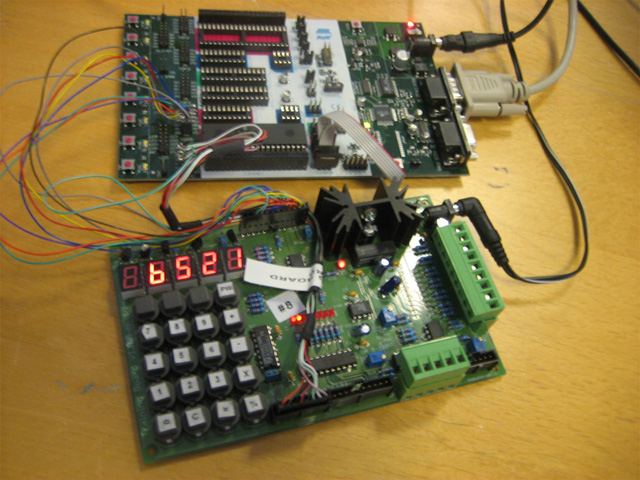## Building the connections

It was necessary to create a custom cable to connect the AVR and the testing board. According with the specification papers the keyboard needs 8 connections (7 data lines and 1 GND) and the LCD needs 11, but as we won’t need brightness control it will be ok with just 10 (9 data and 1 GND). As the AVR ports support just 8 data lines, it’s’ necessary to split the LCD lines into two different ports.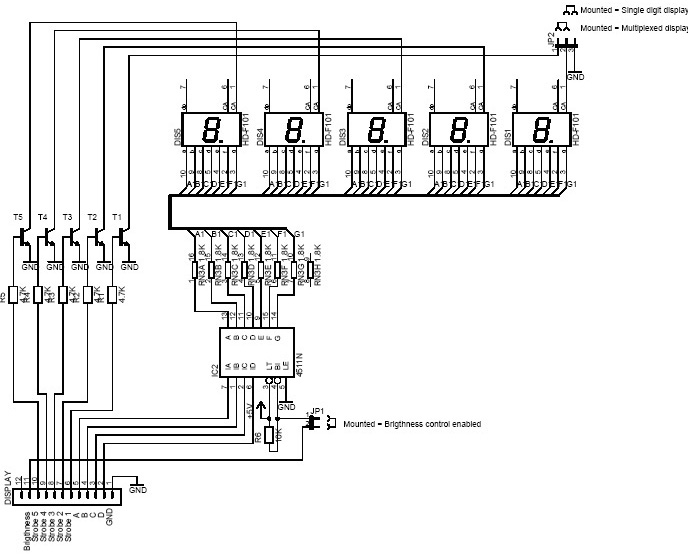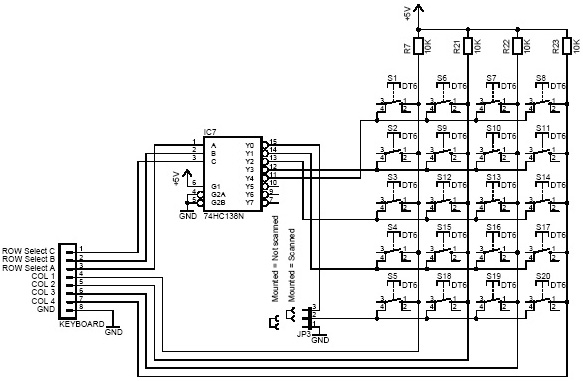Here you can see the final connections reusing one free line from the keyboard port to connect one from of the LCD.## Jumpers in the testing board

• Display:
• JP1: Not connected because we don’t need brightness control. We just use the default brightness for each led.
• JP2: Connected pins 1 and 2 to allow multiplexing display, otherwise we will show just one digit instead of five.
• Keyboard:
• JP3: Connected pins 2 and 3 to allow scanned reading. In that mode we can scan throw all the rows of keys, in the other mode we just scan the first row.

## Workflow

First of all we initialize the hardware setting up all the bits according with the write and read necessities for each port’s pin and setup the default output values for each port (For example not show nothing in the display). After that we initialize the calculator variables that we’re going to use internally to compute the calculator’s operations. Also all the temporary variables such as memory, or last operation or last number. Once we did it we go to the main loop in our program:

• We check if the power button was pressed so the variable power is set to On, if not we wait until we press.
• If power is On we check if there’s some key pressed.
• If there’s some key pressed we get the ID of that key and we send to be processed by the calculator’s main function.

After that we get from the calculator program the number that we need to show and we send to the display function.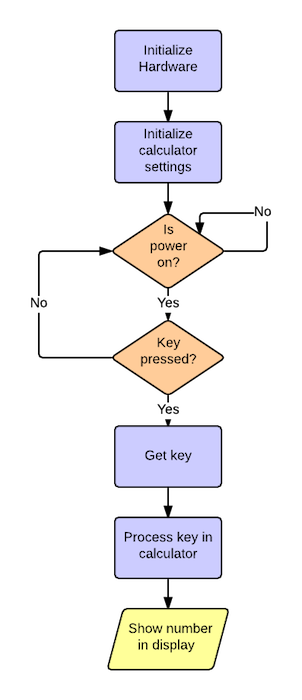## Modules diagram

The program is divided into blocks independent from each others:

• Hardware: Implement all the related to the communication with the hardware in our project. Including:
• Display: Functions to manage the 5 digits diplay.
• Keyboards: Functions to manage the keyboard inputs
• Calculator: The logic in our decimal calculator (Operations, number conversions, etc…)
• Utils: Some util functions such us sleep to use in our program.
• Main: Our main program where is the main workflow detailed before.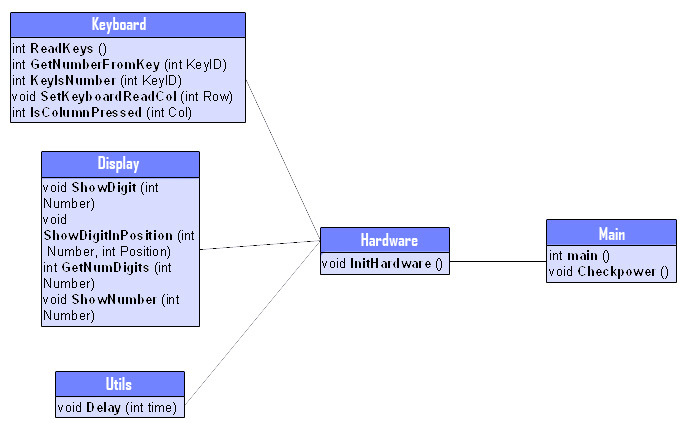## Implementation

### Setting up the hardware

In the call to HardwareInit we need to setup all the bits for start the “dialogue” with our hardware. As we saw in the connection diagrams we’re going to use PortB for display, so we setup that port as use just for output:

``DDRB = 0xff;``

Then we’ll use PortC for Keyboard but we use also the pin6 for display. So we need to put that bit up and also the bits corresponding to row select. These bits (row select) must be set up because we must send which row we want to select to make the scanning to know if was some key pressed in that row.

``DDRC=(1<<PC6)|(1<<PC1)|(1<<PC3)|(1<<PC5);``

### key pressed

Key pressed To know if the user has pressed one key we need to do a scanning throw the keyboard. For that we make a loop along all the rows that we have. We set that row to be the input row (With that we read the information just about that row). For select that row we just need to set the according bits in the Row Select ABC bits. After we look around all the columns if some of these bits are set to up.

``````int ReadKeys()
{
int nRow,nCol;
for (nRow=0; nRow<KEYB_NUM_ROWS; nRow++)
{
for (nCol=0; nCol<KEYB_NUM_COLUMNS; nCol++)
{
if (IsColumnPressed(nCol))
return nRow*10+nCol;
}
}
return -1;
}``````

The code that returns is a two digits identificator for that key. The first digit represent the row and the second the col. Following we have the table of contants for each key value:

CONSTANT VALUE
KEYB_ID_0 0
KEYB_ID_C 1
KEYB_ID_EQUAL 2
KEYB_ID_DIV 3
KEYB_ID_1 10
KEYB_ID_2 11
KEYB_ID_3 12
KEYB_ID_MULT 13
KEYB_ID_4 20
KEYB_ID_5 21
KEYB_ID_6 22
KEYB_ID_MINUS 23
KEYB_ID_7 30
KEYB_ID_8 31
KEYB_ID_9 32
KEYB_ID_CE 40
KEYB_ID_POWER 43

### Show number

The display supports to show one digit in one position at time. It means that is impossible to send one command or set the bits to show more than one in the same time. So what we make is to make a loop throw all the digits that our number has and display in the corresponding position, make a small sleep, and show the next one. With that we make a fast refresh of all the digits so the human’s eye can’t notice the change and you’ll see like is just one number with different digits.

``````void ShowNumber(int nNumber)
{
int nNumDigits,i;
nNumDigits = GetNumDigits(nNumber);
for (i=0; i<nNumDigits; i++)
{
ShowDigitInPosition(nNumber%10, i);
nNumber /= 10;
Delay( 1 );
}
}``````

### Insert new digit in the current number

To insert a new digit to the current number that we’re typing in the display we displace one position left the and add the new number. If the number of digits of the current number if five (the maximum allowed) we just change the last digit of the current number instead of add (Otherwise it will give overflow).

``````void InsertNewDigit(int key)
{
if (GetNumDigits(nCurrentNumber)==5)
nCurrentNumber = 10*(nCurrentNumber/10)+GetNumberFromKey(key);
else
nCurrentNumber = 10*nCurrentNumber+GetNumberFromKey(key);

nShowNumber = nCurrentNumber;
}``````

### Processing key

When we read a key we send the key ID to the function ProcessKey inside it we make a loop waiting for reading while the ID of the read key is the same that we’re going to process:

``while (key==ReadKeys()) {}``

We need to make it because if not it will enter several times in the function if we leave the key pressed.

### Calculate result of the operation

In the calculator we have some global variables:

• nLastAcum: Last accumulator value (Resulting from previous operations).
• nLastOp: Last operation entered by keyboard.
• nShowNumber: Number to show in the screen.
• nCurrentNumber: Current number being typing in the keyboard. Everytime that we press an operation key or the result key we compute the result with the following function:
``````void CalculateResult(int key)
{
nLastAcum = ComputeOperation(nLastAcum,nLastOp,nCurrentNumber);
nLastOp = key;
nShowNumber = nLastAcum;
nCurrentNumber = 0;
}``````